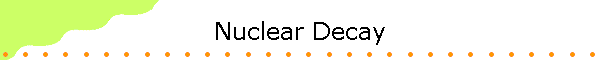Radioactive decay is the set of various processes by which an unstable atomic nucleus emits subatomic particles.  Decay is said to occur in the parent nucleus and produce a daughter nucleus.  The SI unit for measuring radioactive decay is the becquerel (Bq).  If a quantity of radioactive material produces one decay event per second, it has an activity of one Bq.   Since any reasonably-sized sample of radioactive material contains very many atoms, a becquerel is a tiny level of activity.

 The most common decay modes are alpha, beta, and gamma decay. The subatomic particle emitted in alpha decay is a positively charges alpha particle or He nucleus, the subatomic particle emitted in beta decay is a negatively charged electron, and the subatomic particle emitted in gamma decay is neutral photon or gamma ray. We can experimentally identify these particles by passing them through a region containing a magnetic field as shown on the right. The gamma particles carry no charge and are not deflected The alpha particles are deflected upward The beta particles are deflected downward A positron would be deflected upward Link: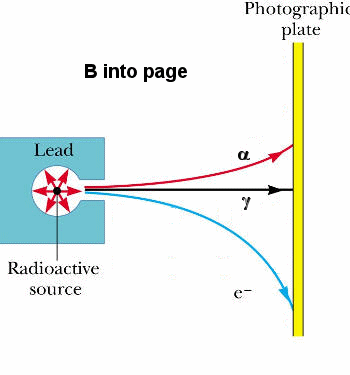The neutrons and protons in a nucleus have various interactions.  The strong nuclear force, not observed at the familiar macroscopic scale, is the most powerful force over subatomic distances.  The electrostatic force is also significant.  Also of importance is the weak nuclear force.  The interplay of these forces is very complex.  If the configuration of the particles in a nucleus shifts ever so slightly, the particles can possibly fall into a lower-energy arrangement.  The nucleus can spontaneously decay.  The resulting transformation changes the structure of the nucleus, it is a nuclear reaction, in contrast to chemical reactions, which concern interactions of electrons with nuclei.

Some nuclear reactions do involve external sources of energy, in the form of "collisions" with outside particles.  However, these are not considered decay.

The decay of an unstable nucleus (radionuclide) is entirely random and it is impossible to predict when a particular nucleus will decay.  It is equally likely to decay at any time.  (If a particular nucleus has a 20% chance to decay in the next day, and it survives for one week, then after that week it still has a 20% chance of decaying in the next day.)  Therefore, given a sample of a particular radioisotope, the number of decay events expected to occur in a small interval of time Δt is proportional to the number N of atoms present.  The decay rate, dN/dt, is proportional to N.  The following first-order differential equation describes the decay.

dN/dt = -λN

Different radionuclides decay at different rates, each having its own decay constant λ.  The negative sign indicates that N decreases with each decay event.  The solution to the above equation is

N(t) = N0exp(-λt).

This function represents exponential decay.  It is an approximate solution, for two reasons.  Firstly, the exponential function is continuous, but the physical quantity N can only take positive integer values.  Secondly, because it describes a random process, it is only statistically true.  However, in most cases, N is a very large number and the function is a good approximation.

In addition to the decay constant λ, radioactive decay is sometimes characterized by the mean lifetime.  Each atom "lives" for a finite amount of time before it decays, and the mean lifetime is the arithmetic mean of all the atoms' lifetimes.  It is represented by the symbol τ, and is related to the decay constant by

τ = 1/λ.

The probability that a nucleus will decay in the next time interval dt is λdt.  The probability that a nucleus present at t = 0 will still be present at time t is N(t)/N0 = exp(-λt).  The probability that it will decay in the time interval between t and t + dt is exp(-λt)λdt.  The mean lifetime of a nucleus therefore is

< t > = τ = ∫0t exp(-λt)λdt  = λ∫0t exp(-λt)dt = 1/λ.

[The integral is evaluated using integration by parts.
d(uv) = udv + vdu.  Let u = t and v = -exp(-λt)/λ, then du = dt and dv = exp(-λt)dt

-d(t exp(-λt)/λ) = t exp(-λt)dt  - (1/lλ)exp(-λt)dt.

0t exp(-λt)dt = (1/λ)∫0exp(-λ)dt  -  ∫0d(t exp(-λt)/λ) = (-1/λ2)exp(-λt)|0 - t exp(-λt)/λ|0 = -1/λ2.]

A commonly used parameter is the half-life t1/2.  Given a sample of a particular radionuclide, the half-life is the time it takes for half the radionuclides to decay.  In terms of τ the half life is given by

t1/2 = τ ln2 = ln2/λ.

[N(t)/N0 = exp(-λt) = 1/2.  λt = ln2.  t = ln2/λ.]

Highly radioactive substances are quickly lost, while those that radiate weakly endure longer.  Half-lives of known radionuclides vary widely, from 109 years for nearly stable nuclides, to 10-6 seconds for highly unstable nuclides.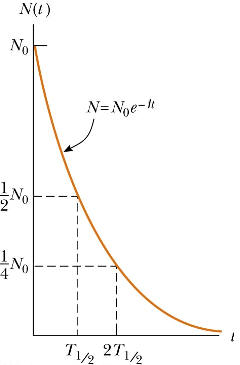We are often more interested in the decay rate R = -dN/dt than in the number N itself.

N(t) = N0exp(-λt),  R  = -dN/dt = λN0exp(-λt) = R0exp(-λt), R0 = λN0.

Problem:

A 2.71 g sample of KCl is found to be radioactive.  It is decaying at a rate of 4490 Bq.  The decay is traced to an isotope of potassium, 40K, which constitutes 1.17% of normal potassium.  Calculate the half life of this nuclide.

• Solution:
t1/2 = ln2/λ.  R0 = λN0.  t1/2 = N0ln2/R0.
R0 = 4490/s,  we need to find N0.
There are an equal number of K and Cl atoms in the sample.
The number of K atoms in the sample is equal to the number of moles in the sample * NA.
Number of moles in the sample =( mass of sample)/(molar mass of KCl).
Using an atomic and nuclear data table we find the molar mass of potassium to be 39.098 g/mole and the molar mass of chlorine to be 35.453 g/mole, so the molar mass of KCl is 74.552 g/mole.
Therefore the number of 40K nuclei in the sample is N0 = 0.0117*NA*2.71/74.552 = 2.56*1020.
t1/2 = (2.56*1020*ln2/4490)s = 3.95*1016 s = 1.25*109 years.

α-decay

Alpha decay is a form of radioactive decay in which an atomic nucleus characterized by mass number A and atomic number Z ejects an alpha particle and transforms into a nucleus with mass number A - 4 and atomic number Z - 2 .

Example:

Thus the most common form of Uranium, 238U (A = 238, Z = 92) goes to Thorium (A = 234, Z = 90) by α -decay.

238U --> 234Th + α

The surprising thing is that for this process τ is  comparable to the age of the universe.  If the decay is energetically desirable, why does it take so long?  The answer is that there exists a potential barrier.  In a simple model the α-particle exists inside the U nucleus, confined in a deep square well potential representing the attractive nuclear force.  However, the α-particle wave function penetrates some distance into the wall of this finite square well potential, where the α-particle will be out of the range of the nuclear force, but not out of the range of the long-range electrostatic repulsive force of the other protons in the nucleus.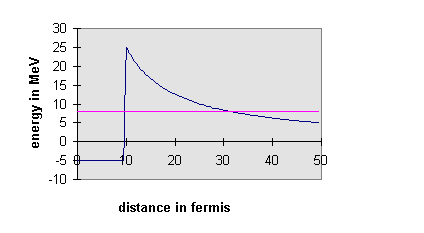If the total energy of the α-particle inside the well is larger than its potential energy outside the well, the a-wave function can re-emerge oscillating at some distance.  There is some finite probability of finding the α-particle shooting away from the nucleus.  The actual probability of this happening depends on how much the wave function decays as it tunnels through the barrier, which depends on the total energy of the particle.  For 238U, the wave function decays so much that that the α-decay has a half-life of over a billion years.  However, many different nuclei undergo α-decay, the quickest in millionths of a second.  The energies of the emitted α-particles can be measured, and the measurements confirm the explanation of quantum mechanical tunneling, first put forward in 1928.  The quickest decays correspond to the most energetic α-particles, just as the tunneling picture would suggest.

Note that an alpha particle is a helium nucleus, and that both mass number and atomic number are conserved in the decay.  Alpha decay can essentially be thought of as nuclear fission where the parent nucleus splits into two daughter nuclei.  Alpha decay is fundamentally a quantum tunneling process.  In some radioactive substances, when beta decay occurs along with alpha decay, atomic helium is formed.  Alpha particles or alpha rays are a form of particle radiation which are highly ionizing and have low penetration.

Most alpha particles are emitted with approximately 5 MeV of kinetic energy.  Virtually all of the helium produced in the United States and elsewhere comes from trapped underground deposits associated with Uranium- or Thorium-containing minerals which alpha decay.  The Helium is brought to the surface as a by-product of natural gas production.

Problem:

Calculate the energy Q released during the alpha decay 238U --> 234Th + 4He.
The atomic masses are:

 238U 238.05079 u 234Th 234.04363 u 4He 4.00260 u
• Solution:
Q = mic2 - mfc2
mi = initial mass, mf = final mass
mi - mf = (238.05079 - (234.04363 + 4.00260)) u = 0.00456 u
(The electron masses which are included in the atomic masses cancel out.)
Q = (0.00456 u)c2*(931.494 MeV/c2)/u) = 4.25 MeV

Show that 238U cannot spontaneously emit a proton.

• Solution:
238U --> 237Pa + 1H.  m(237Pa) = 237.05121 u, m(1H) = 1.00783 u.
Q/c2 = 238.05079 u - 237.05121 u - 1.00783 u = - 0.00825 u.
The energy released is negative, we have to add energy to make this reaction possible.

In-class activityAlpha decay

β-decay

Beta decay (sometimes called neutron decay) is a type of radioactive decay in which a β-particle or electron is emitted.  A neutron alone in space is unstable.  It will only last about ten minutes before ejecting an electron and leaving a proton behind.  This is energetically possible, because the mass of the neutron exceeds the sum of the masses of the proton and electron.  The process is called β-decay.

Heavier nuclei have more neutrons than protons, because the Coulomb repulsion makes it harder to bind protons.  But neutrons are fermions and the Pauli exclusion principle requires that as we add more and more neutrons, the energy of the added neutrons will be higher and higher.  So β-decay is often favored energetically.

In β decay, the weak nuclear interaction converts a neutron into a proton while emitting an electron and an anti-neutrino.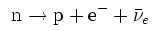In β+ decay, a proton is converted into a neutron, a positron and a neutrino.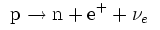If the proton and neutron are part of an atomic nucleus the decay processes transmute one chemical element into another.

Example: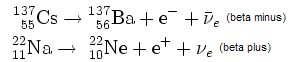Historically, the study of beta decay provided the first physical evidence of the neutrino.  The energies of electrons emitted in beta decay were observed to be non-discrete.  Some electrons were more energetic than others.  A problem arose in trying to explain what had happened to the missing energy if an electron was emitted with less than the maximum energy available.  Conservation of energy appeared to be violated.  Wolfgang Pauli proposed that the "missing" energy was actually carried away by another, yet undiscovered, particle.  This was analyzed in more detail by Enrico Fermi.  Fermi called the particle the neutrino, the little neutral one.

Detecting neutrinos is still difficult, since they are not sensitive to the strong nuclear force or to electromagnetic fields.  The force that causes β-decay is called the weak force.  The only way to detect a neutrino is when it collides with a particle and interacts weakly, essentially reversing one of the β-decays.  A neutrino is extremely unlikely to interact with a nucleus, in fact a neutrino will almost certainly just pass through you.  It takes large detectors and a strong flux of neutrinos to detect neutrinos.  Much effort is being currently expended detecting neutrinos from the sun, since they are our only window into the nuclear processes taking place deep in the sun.  They can escape without interacting with the outer layers, unlike photons or any other particle emitted.

In the radioactive decay of 238U it emits an α-particle to become 234Th, then β-decays twice in succession to become 234U.  This nucleus is now relatively proton-rich, and it α-decays five times in succession to become a form of lead,

234U --> 230Th --> 226Ra --> 222Rn --> 218Po --> 214Pb

214Pb undergoes two β-decay, an α-decay, two β-decays, and another α-decay, before it reaches stability at 206Pb.  The sequence above is through metallic elements with one exception, Radon (Rn).  Radon is a noble gas and so it is chemically inert.  It emits its α-particle in a period of days, which gives it time to diffuse out of the rock into somebody’s basement.

 214Pb β- 214Bi 214Bi β- (99.98%) 214Po 214Po α 210Pb 210Pb β- 210Bi 210Bi β- (99.99987%) 210Po 210Po α 206Pb 206Pb -

Simulation:  Beta decay

Problem:

Calculate the disintegration energy Q for the beta decay 32P --> 32S + e- + ν.
Atomic masses:  m(32P) = 31.97391 u, m(32S) = 31.97207 u

• Solution:
Q = mic2 - mfc2
mi = mnuc(32P) = m(32P) - 15*m(e-),
mf = mnuc(32S) + m(e-) = m(32S) - 16*m(e-) + m(e-) = m(32S) - 15*m(e-)
The mass of the neutrino is negligibly small.
Q = mic2 - mfc2 = (m(32P) - m(32S))c2 = 1.71 MeV.
In β- decay subtracting the atomic masses automatically takes into account the mass of the emitted electron.  This is not true for β+ decay.

Calculate the disintegration energy Q for the beta decay 64Cu --> 64Ni + e+ + ν.
Atomic masses:  m(64Cu) = 63.929766 u, m(64Ni) = 63.927968 u

• Solution:
Q = mic2 - mfc2
mi = mnuc(64Cu) = m(64Cu) - 29*m(e-),
mf = mnuc(64Ni) + m(e+) = m(64Ni) - 28*m(e-) + m(e+) = m(64Ni) - 27m(e-)
The mass of the neutrino is negligibly small and  m(e+) =  m(e-).
Q = mic2 - mfc2 = (m(64Cu) - m(64Ni))c2 - 2*m(e-)c2 = 1.6748 MeV - 2*0.511 MeV
= 0.653 MeV.
In β+ decay subtracting the atomic masses does not automatically take into account the mass of the emitted positron.

γ-decay

The three forms of radiation emitted by decaying nuclei, α , β and γ , were named before the particles being emitted were fully identified.  It was only realized later that the β-rays were electrons.  The γ-rays are just high-energy photons, of order 100 keV to a few MeV.  Emission of γ rays is similar to emission of photons by excited states of atoms.  The nucleus can be excited by having just emitted an α- or β-particle, or by colliding with another nucleus, or by being bombarded by neutrons.  All these events can lead to a nucleus which is excited, and electromagnetic radiation is emitted.

Photons with energies of more than 10 keV are often called gamma rays, although electromagnetic radiation from around 10 keV to several hundred keV is also referred to as hard x-rays.  There is no physical difference between gamma rays and x-rays of the same energy, these are two names for the same electromagnetic radiation.  Rather, gamma rays are distinguished from x-rays by their origin.  Gamma ray is a term for high-energy electromagnetic radiation produced by nuclear transitions, while x-ray is a term for high-energy electromagnetic radiation produced by energetic electrons.  Because it is possible for some electron transitions to be of higher energy than some nuclear transitions, there is an overlap between what we call low energy gamma rays and high energy x-rays.

Gamma rays are a form of ionizing radiation, they are more penetrating than either alpha- or beta-radiation, but less ionizing.